## IDENTIFICATION OF PIPE CROSS-SECTION

If flow rate is stable in water flow way, continuity balance is maintained.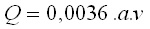Q : Load amount ( m3/h )
a : Pipe cross section ( mm2 )
v : Flow rate ( m/s )

To calculate the necessary pipe cross-section, below practical and useful formulae is used: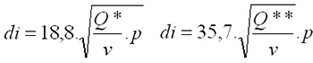di : Pipe Internal Diameter ( mm )
Q* : Load amount ( m3/h )
Q** : Load amount ( l/h)

## PRESSURE LOSSES

Below values substantially effects the hydraulic pressure losses:

• Length of Pipe line
• Pipe diameter of straight line
• Pipe smoothness
• Pipe connections (fittings and armatures)
• Fluid density
• Flow form (regular or irregular flow)

TTotal pressure losses are the sum of separate pressure losses as stated below: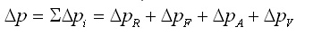### Calculation of Separate pressure losses

* Pressure loss in the straight pipe line DpR

Below formulae is used for High energy loss (hv) or pressure loss (Dp) due to decreasing flow amount, flow rate and pressure decrease in the HDPE Pipes.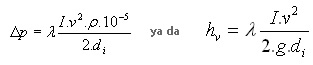di : Pipe Internal Diameter (mm)
I : Length of pipe line (mm)
v : Average fluid flow rate (m/h)
p : fluid density (kg/m3)
l : Friction co-efficient (0.02 sufficient)
g : Gravity (9,81 m/s2)

High energy loss means the height differences on the slopes in order to obtain desired flow rates in the line.Friction co-efficient I is under the scope of general formulae below: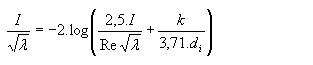Re : Reynold number (v.d/v)
o : Kinematic fluidity of water ( 1.31.16-6 m²/s )
k :Hydraulic smoothness value of pipe internal surface (m)

Below table presents smoothness k values for various pipe types.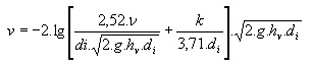Line Type Smoothness k (mm)
Steel, new 0,01 .... 0,1
Ductile pipe, new 0,0001 .... 1
Ductile pipe, old 0,03 .... 0,2
HDPE - FCS 0,007 .... 0,015
Concrete pipe, new 1,0 .... 2,0
Ceramics pipe 0,1 .... 1,0
Old pipe 2,0

### Kb value that defines the service smoothness:

• Wall smoothness
• Straightness of Pipe line
• Water-hammer
• Manholes
• Inlet and Outlet storages

### Pressure losses in Fittings DpF: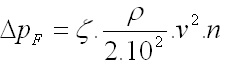ζ : Fitting resistance value
p : Fluid density (kg/m3)
v : Flow rate (m/h)
n : Number of Fittings

### Pressure losses in armatures DpA :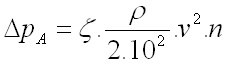ζ : Armature Resistance
p : Fluid density (kg/m3)
v : Flow rate (m/h)
n : Number of Fittings

Resistance value of armatures is between 0.5 and 5.0. This value is known by the armature producer.

### Pressure losses in the pipe connections Dpv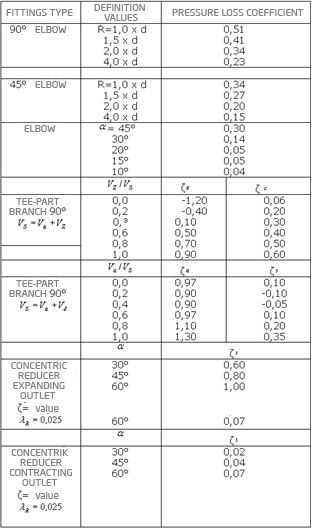Positive ζ Value : Pressure decrease
Negative ζ Value : Pressure increase
va : Inlet flow rate
vd : Outlet flow rate
vs : Total inlet flow rate
vx : Fitting inlet flow rate

## WATER-HAMMERING

During opening/shutting the valve or pump, water-hammer may occur in the line. For this, theoretically, greater ps:

a: Spread rate of pressure wave (m/h)
v0: Flow rate of fluid (m/h)
p: Fluid density (kg/m3)